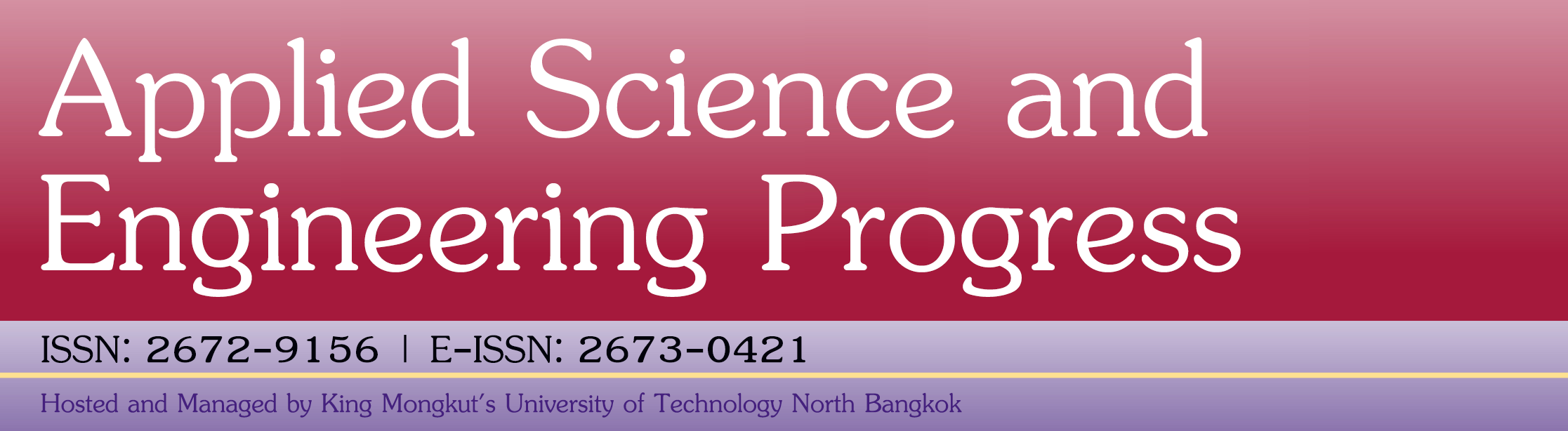Finding a Best Fit Plane to Non-coplanar Point-cloud Data Using Non Linear and Linear Equations

Mulay A., Ahuja B.B.

Abstract

In recent times, dimensional model fitting has gained wide acceptance in various fields of manufacturing including co-ordinate metrology, reverse engineering and computer / machine vision. In this paper, a methodology of least square technique is discussed which can be used to find the equation of a best fit plane for non-coplanar points obtained by laser scanning of an object. The theoretical method to get the equation of best fit plane includes a solution of non-linear homogeneous set of equations which are not easy to tackle and as such some method is to be developed to convert the non-linear set of homogeneous set of equations into linear simultaneous equations. A case study is also introduced to explain the procedure to handle linear as well as non-linear simultaneous equations. These set of linear simultaneous equations can then be used in various applications like fast surface generation in reverse engineered projects and even in the field of computer aided inspection for surface roughness measurement using light sectioning vision system where similar approach can be used.

Full Text: PDF

Refbacks

• There are currently no refbacks.